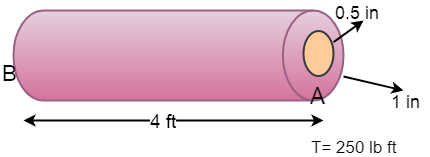# Determine the angle of twist ( ? ) of point A of the rod subjected to the torque shown. The rod...

## Question:

Determine the angle of twist ({eq}\phi {/eq}) of point A of the rod subjected to the torque shown. The rod is fixed at B and is made of a steel outer tube (G = {eq}11.4\times 10^{3} kips/in^{2} {/eq}) and a solid brass inner core(G = {eq}5.2\times 10^{3} kips/in^{2} {/eq}). The overall radius of the rod is 1 inch, and the radius of the brass core is 0.5 inch.## Angle of twist

When the shaft is subjected to external torque, then it gets twisted. The angle through which one end of shaft twists relative to the other end is known as angle of twist.

Become a Study.com member to unlock this answer! Create your account

Given Data

• The modulus of rigidity of the steel tube is: {eq}{G_{st}} = 11.4 \times {10^3}\;{{{\rm{kips}}} {\left/ {\vphantom {{{\rm{kips}}}...Torque: Concept, Equation & Example

from

Chapter 7 / Lesson 4
13K

After watching this lesson, you will be able to explain what torque is conceptually and mathematically and use both the equation and Newton's First Law for rotation to solve problems involving torque. A short quiz will follow.